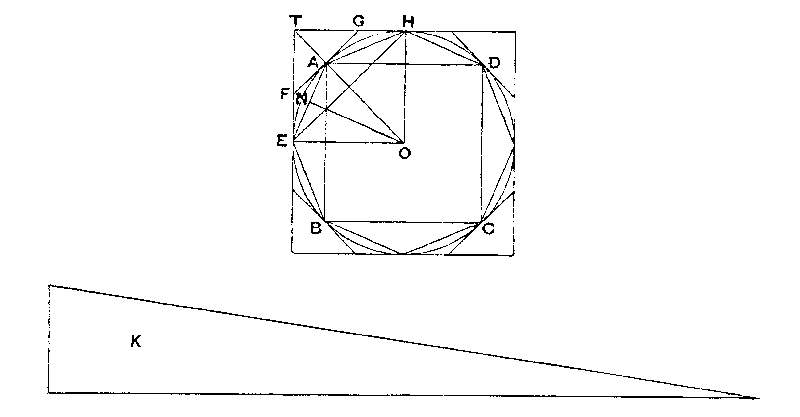MEASUREMENT OF A CIRCLE.

Proposition 1.
The area of any circle is equal to a right-angled triangle in which one of the sides about the right angle is equal to the radius, and the other to the circumference, of the circle.Let ABCD be the given circle, K the triangle described
Then, if the circle is not equal to K, it must be either greater or less.
I. If possible, let the circle be greater than K.
Inscribe a square ABCD, bisect the arcs AB, BC, CD, DA, then bisect (if necessary) the halves, and so on, until the sides of the inscribed polygon whose angular points are the points of division subtend segments whose sum is less than the excess of the area of the circle over

Thus the area of the polygon is greater than K.

Let AE be any side of it, and ON the perpendicular on AE from the centre 0.

Then ON  is less than the radius of the circle and therefore less than one of the sides about the right angle in K. Also the perimeter of the polygon is less than the circumference of the circle, i.e.  less than the other side about the right angle in K.

Therefore the area of the polygon is less than K; which is inconsistent with the hypothesis

Thus the area of the circle is not greater than.

II. If possible, let the circle be less than K

Circumscribe a square, and let two adjacent sides, touching the circle in E, H, meet in T.  Bisect the arcs between adjacent points of contact and draw the tangents at the points of bisection. Let A be the middle point of the arc EH, and FAG the tangent at A.

Then the angle TAG is a right angle.
Therefore                                     TG > GA

> GH.

It follows that the triangle FTG is greater than half the area TEAH.

Similarly, if the arc AH be bisected and the tangent at the point of bisection be drawn, it will cut off from the area GAH more than one-half.

Thus, by continuing the process, we shall ultimately arrive at a circumscribed polygon such that the spaces intercepted between it and the circle are together less than the excess of K over the area of the circle.

Thus the area of the polygon will be less than K

Now, since the perpendicular from 0 on any side of the polygon is equal to the radius of the circle, while the perimeter of the polygon is greater than the circumference of the circle, it follows that the area of the polygon is greater than the triangle K; which is impossible.

Therefore the area of the circle is not less than K.

Since then the area of the circle is neither greater nor less than K, it is equal to it.

Proposition 2.
The area of a circle is to the square on its diameter as 11 to 14
[The text of this proposition is not satisfactory, and Archimedes cannot have placed it before Proposition 3, as the approximation depends upon the result of that proposition.]

Proposition 3.
The ratio of the circumference of any circle to its diameter is less than  3 1/7  but greater than  3 10/71.